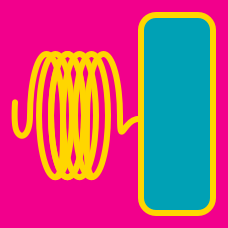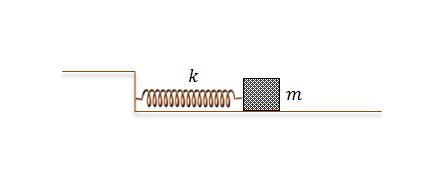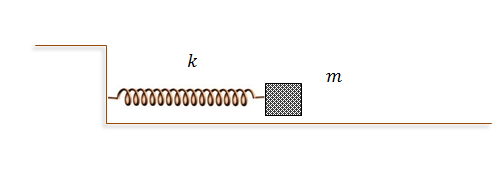Classical Mechanics

# Damped Oscillators - Problem SolvingA body of mass $m = 1 \text{ kg}$ is oscillating on a spring with spring constant $k = 2 \text{ N/m}.$ There is a friction proportional to the body's velocity, represented by the function $f = -bv = -2v \text{ (N)}$. Initially, the position of the body was $x(t=0) = 2 \text{ m }$ and the velocity was $v(t=0) = 0 \text{ m/s },$ where $x$ denotes the difference in the length of the spring from its original length. What is the amplitude of the body when $t = 2 \text{ s } ?$

Assume that $\sqrt{2} = 1.5$A body of mass $m = 2 \text{ kg}$ is oscillating on a spring with spring constant $k = 4 \text{ N/m}.$ There is a friction proportional to the body's velocity, represented by the function $f = -bv = -4v \text{ (N)}$. Initially, the position of the body was $x(t=0) = 5 \text{ m }$ and the velocity was $v(t=0) = 0 \text{ m/s },$ where $x$ denotes the difference in the length of the spring from its original length. What is the velocity of the body when $t = \frac{\pi}{2} \text{ s } ?$A body of mass $m = 3 \text{ kg}$ is oscillating on a spring with spring constant $k = 3 \text{ N/m}.$ There is a friction proportional to the body's velocity, represented by the function $f = -bv = -6v \text{ (N)}.$ Initially, the position of the body was $x(t=0) = 1 \text{ m }$ and the velocity was $v(t=0) = 0 \text{ m/s },$ where $x$ denotes the difference in the length of the spring from its original length. What is the velocity of the body when $t = 1 \text{ s } ?$A body of mass $m = 6 \text{ kg}$ is oscillating on a spring with spring constant $k = 12 \text{ N/m}.$ There is a friction proportional to the body's velocity, represented by the function $f = -bv = -12v \text{ (N)}.$ Initially, the position of the body was $x(t=0) = 4 \text{ m }$ and the velocity was $v(t=0) = 0 \text{ m/s },$ where $x$ denotes the difference in the length of the spring from its original length. What is the ratio of the amplitude to the initial amplitude when $t = 5 \text{ s } ?$A body of mass $m = 3 \text{ kg}$ is oscillating on a spring with spring constant $k = 3 \text{ N/m}.$ There is a friction proportional to the body's velocity, represented by the function $f = -bv = -6v \text{ (N)}$. Initially, the position of the body was $x(t=0) = 3 \text{ m }$ and the velocity was $v(t=0) = 0 \text{ m/s },$ where $x$ denotes the difference in the length of the spring from its original length. What is the position of the body when $t = 3 \text{ s } ?$

×